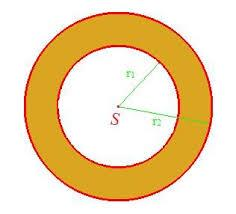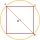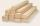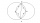# Circular ring

Square with area 16 centimeters square are inscribed circle k1 and described circle k2. Calculate the area of circular ring, which circles k1, k2 form.

Correct result:

S =  12.5664 cm2

#### Solution:We would be pleased if you find an error in the word problem, spelling mistakes, or inaccuracies and send it to us. Thank you!Tips to related online calculators
Pythagorean theorem is the base for the right triangle calculator.

#### You need to know the following knowledge to solve this word math problem:

We encourage you to watch this tutorial video on this math problem:

## Next similar math problems:

• Center traverseIt is true that the middle traverse bisects the triangle?The double ladder shoulders should be 3 meters long. What height will the upper top of the ladder reach if the lower ends are 1.8 meters apart?The double ladder is 8.5m long. It is built so that its lower ends are 3.5 meters apart. How high does the upper end of the ladder reach?
• Tree trunkWhat is the smallest diameter of a tree trunk that we can cut a square-section square with a side length of 20 cm?
• SquareCalculate area of the square with diagonal 64 cm.
• LogsThe log has diameter 30 cm. What's largest beam with a rectangular cross-section can carve from it?
• Diamond area from diagonalsIn the diamond ABCD is AB = 4 dm and the length of the diagonal is 6.4 dm long. What is the area of the diamond?
• RT - inscribed circleIn a rectangular triangle has sides lengths> a = 30cm, b = 12.5cm. The right angle is at the vertex C. Calculate the radius of the inscribed circle.
• Isosceles trapezoidCalculate the area of an isosceles trapezoid whose bases are in the ratio of 4:3; leg b = 13 cm and height = 12 cm.
• Isosceles triangleCalculate the area of an isosceles triangle, the base of which measures 16 cm and the arms 10 cm.
• Equilateral triangleThe equilateral triangle has a 23 cm long side. Calculate its content area.
• Tree trunkFrom the tree trunk, the diameter at the narrower end is 28 cm, a beam of square cross-section is to be made. Calculate the longest side of the largest possible square cross-section.
• Square diagonalCalculate the length of diagonal of the square with side a = 23 cm.
• Annular areaThe square with side a = 1 is inscribed and circumscribed by circles. Find the annular area.
• Two circlesTwo circles with the same radius r = 1 are given. The center of the second circle lies on the circumference of the first. What is the area of a square inscribed in the intersection of given circles?
• WasteHow many percents are waste from a circular plate with a radius of 1 m from which we cut a square with the highest area?
• Company logoThe company logo consists of a blue circle with a radius of 4 cm, which is an inscribed white square. What is the area of the blue part of the logo?